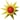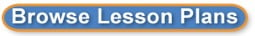Search results for Algebra 1:
 Browse All Lesson Plans Lesson Plan Name Grades Linear Relationships in the Real World 9 to 12(0 stars, 1 ratings)The student will use problem solving, mathematical communication, mathematical reasoning, connections and representations to solve multi-step equations in one variable with the variable on one of two sides of the equations while identifying at least 3 careers which utilize this skill. Graphing quadratic equations of the form f(x) = ax^2 + c 9 to 12(0 stars, 1 ratings)In this lesson, we explore the effect of the constant C in the quadratic function f(x) = ax^2. Students will be able to observe that C shifts the quadratic function up/down. Interpret the equation 8 to 8(0 stars, 1 ratings)To interpret the equation of line, students will rotate through three stations. Each station will require the students to interpret the equation but using different techniques. Vocabulary Taboo Review 3 to 12(0 stars, 1 ratings)This lesson is a twist on the card game "Taboo", where people aim to describe a word at the top of the card, but are not allowed to use the "Taboo" words printed on the card in their description.